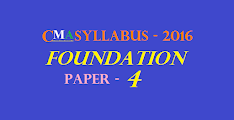• CURRENT EVENTS

Welcome to Our new blog especially for CMA, CA and CS Exam.PAPER – 4 : FUNDAMENTALS OF BUSINESS MATHEMATICS AND STATISTICS
 Section A : Fundamentals of Business Mathematics Section B : Fundamentals of Business Statistics 1. Arithmetic 20% 2. Algebra 20% 3. Statistical representation of Data 10% 4. Measures of Central Tendency and Dispersion 30% 5. Correlation and Regression 10% 6. Probability 10%
Section A : Fundamentals of Business Mathematics [40 Marks]
1. Arithmetic
(a) Ratios, Variations and Proportions
(b) Simple and Compound interest
(c) Arithmetic Progression and Geometric Progression.
2. Algebra
(a) Set Theory
(b) Indices and Logarithms (basic concepts)
(c) Permutation and Combinations (basic concepts)
(d) Quadratic Equations (basic concepts)
Section B : Fundamentals of Business Statistics [60 Marks]
3. Statistical Representation of Data
(a) Diagrammatic representation of data
(b) Frequency distribution
(c) Graphical representation of Frequency Distribution – Histogram, Frequency Polygon Curve, Ogive, Pie-chart
4. Measures of Central Tendency and Dispersion
(a) Mean, Median, Mode, Mean Deviation
(b) Range, Quartiles and Quartile Deviation
(c) Standard Deviation
(d) Co-efficient of Variation
(e) Karl Pearson and Bowley’s Co-efficient of Skewness
5. Correlation and Regression
(a) Scatter diagram
(b) Karl Pearson’s Coefficient of Correlation
(c) Regression lines, Regression equations, Regression coefficients
6. Probability
(a) Independent and dependent events; Mutually exclusive events
(b) Total and Compound Probability; Baye’s theorem; Mathematical Expectation